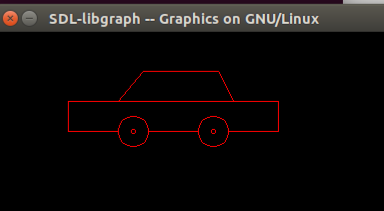# Draw a moving car using computer graphics programming in C

• Difficulty Level : Medium
• Last Updated : 07 Oct, 2021

In computer graphics, use graphics.h which provide direct functions to draw different coordinate shapes (like circle, rectangle etc). By using these functions we can draw different objects like car, hut, trees, etc. In this program, we will draw a moving car using line and circles.

Functions used in program:

• delay(n): This function is used for holding the program output for a small period of time since processing is very fast so use it to see the result.
• setcolor(n): A function from graphics.h header file which set the color of the pointer (cursor). There are some predefined colors in computer graphics. Here n is color number.
• line(x1, y1, x2, y2): A function from graphics.h header file which draw a line with (x1, y1) as first coordinate of line and (x2, y2) as second coordinate of the line.
• circle(x, y, r): A function from graphics.h header file which draw a circle with center (x, y) and radius r.

Example 1: This example create a moving car without using cleardevice() method.

## C++

 `// C program to draw a moving car. This` `// program run in gcc compiler having` `// graphics.h library installed` `#include ` `#include `   `// Function to draw moving car` `void` `draw_moving_car(``void``) {`   `    ``int` `i, j = 0, gd = DETECT, gm;`   `    ``// Passed three arguments to initgraph` `    ``// function to initialize graphics mode` `    ``initgraph(&gd, &gm, ``""``);`   `    ``for` `(i = 0; i <= 420; i = i + 10) {`   `        ``// Set color of car as red` `        ``setcolor(RED);`   `        ``// Thease lines for bonnet and ` `        ``// body of car` `        ``line(0 + i, 300, 210 + i, 300);` `        ``line(50 + i, 300, 75 + i, 270);` `        ``line(75 + i, 270, 150 + i, 270);` `        ``line(150 + i, 270, 165 + i, 300);` `        ``line(0 + i, 300, 0 + i, 330);` `        ``line(210 + i, 300, 210 + i, 330);`   `        ``// For left wheel of car` `        ``circle(65 + i, 330, 15);` `        ``circle(65 + i, 330, 2);`   `        ``// For right wheel of car` `        ``circle(145 + i, 330, 15);` `        ``circle(145 + i, 330, 2);`   `        ``// Line left of left wheel` `        ``line(0 + i, 330, 50 + i, 330);`   `        ``// Line middle of both wheel` `        ``line(80 + i, 330, 130 + i, 330);`   `        ``// Line right of right wheel` `        ``line(210 + i, 330, 160 + i, 330);`   `        ``delay(100);`   `        ``// To erase previous drawn car, draw` `        ``// the whole car at same position` `        ``// but color using black` `        ``setcolor(BLACK);` `        `  `        ``// Lines for bonnet and body of car` `        ``line(0 + i, 300, 210 + i, 300);` `        ``line(50 + i, 300, 75 + i, 270);` `        ``line(75 + i, 270, 150 + i, 270);` `        ``line(150 + i, 270, 165 + i, 300);` `        ``line(0 + i, 300, 0 + i, 330);` `        ``line(210 + i, 300, 210 + i, 330);`   `        ``// For left wheel of car` `        ``circle(65 + i, 330, 15);` `        ``circle(65 + i, 330, 2);`   `        ``// For right wheel of car` `        ``circle(145 + i, 330, 15);` `        ``circle(145 + i, 330, 2);`   `        ``// Line left of left wheel` `        ``line(0 + i, 330, 50 + i, 330);`   `        ``// Line middle of both wheel` `        ``line(80 + i, 330, 130 + i, 330);`   `        ``// Line right of right wheel` `        ``line(210 + i, 330, 160 + i, 330);` `    ``}`   `    ``getch();`   `    ``closegraph();` `}`   `// Driver code` `int` `main()` `{` `    ``draw_moving_car();`   `    ``return` `0;` `}`

Output:Example 2: This example uses cleardevice() method to clear the screen.

## C

 `// C program to draw a moving car. This` `// program run in gcc compiler having` `// graphics.h library installed` `#include ` `#include `   `// Function to draw moving car` `void` `draw_moving_car(``void``) {`   `    ``int` `i, j = 0, gd = DETECT, gm;`   `    ``// Passed three arguments to initgraph` `    ``// function to initialize graphics mode` `    ``initgraph(&gd, &gm, ``""``);`   `    ``for` `(i = 0; i <= 420; i = i + 10) {`   `        ``// Set color of car as red` `        ``setcolor(RED);`   `        ``// Thease lines for bonnet and ` `        ``// body of car` `        ``line(0 + i, 300, 210 + i, 300);` `        ``line(50 + i, 300, 75 + i, 270);` `        ``line(75 + i, 270, 150 + i, 270);` `        ``line(150 + i, 270, 165 + i, 300);` `        ``line(0 + i, 300, 0 + i, 330);` `        ``line(210 + i, 300, 210 + i, 330);`   `        ``// For left wheel of car` `        ``circle(65 + i, 330, 15);` `        ``circle(65 + i, 330, 2);`   `        ``// For right wheel of car` `        ``circle(145 + i, 330, 15);` `        ``circle(145 + i, 330, 2);`   `        ``// Line left of left wheel` `        ``line(0 + i, 330, 50 + i, 330);`   `        ``// Line middle of both wheel` `        ``line(80 + i, 330, 130 + i, 330);`   `        ``// Line right of right wheel` `        ``line(210 + i, 330, 160 + i, 330);`   `        ``delay(100);`   `        ``// To erase previous drawn car` `        ``// use cleardevice() function` `        ``cleardevice();` `    ``}`   `    ``getch();`   `    ``closegraph();` `}`   `// Driver code` `int` `main()` `{` `    ``draw_moving_car();`   `    ``return` `0;` `}`

Output:My Personal Notes arrow_drop_up
Recommended Articles
Page :